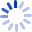# Pattern Recognition and Machine Learning

This is the first textbook on pattern recognition to present the Bayesian viewpoint. It presents approximate inference algorithms that permit fast approximate answers in situations where exact answers are not feasible, and it uses graphical models to describe probability distributions.

##### Cash for Textbooks